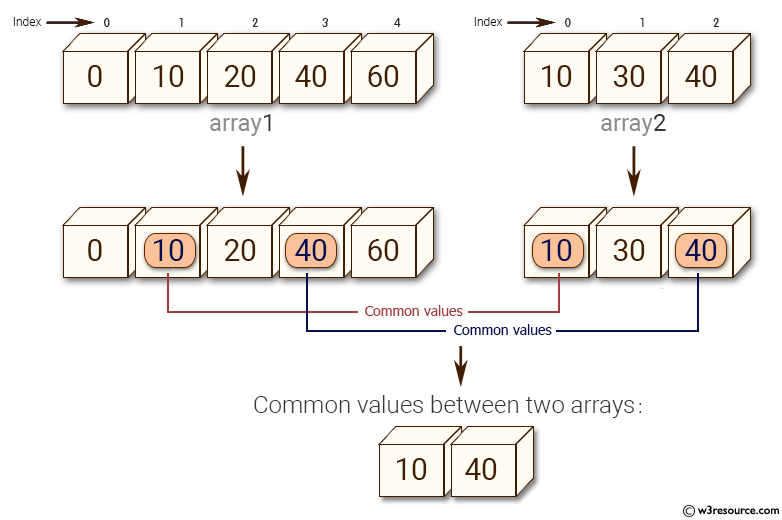﻿ NumPy: Find common values between two arrays - w3resource# NumPy: Find common values between two arrays

## NumPy: Array Object Exercise-18 with Solution

Write a NumPy program to find common values between two arrays.

Pictorial Presentation:Sample Solution:-

Python Code:

``````import numpy as np
array1 = np.array([0, 10, 20, 40, 60])
print("Array1: ",array1)
array2 = [10, 30, 40]
print("Array2: ",array2)
print("Common values between two arrays:")
print(np.intersect1d(array1, array2))
```
```

Sample Output:

```Array1:  [ 0 10 20 40 60]
Array2:  [10, 30, 40]
Common values between two arrays:
[10 40]
```

Python Code Editor:

Have another way to solve this solution? Contribute your code (and comments) through Disqus.

What is the difficulty level of this exercise?

Test your Python skills with w3resource's quiz

﻿

## Python: Tips of the Day

Set comprehension:

```>>> m = {x ** 2 for x in range(5)}
>>> m
{0, 1, 4, 9, 16}
```